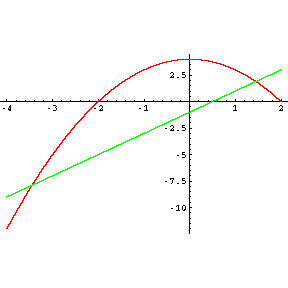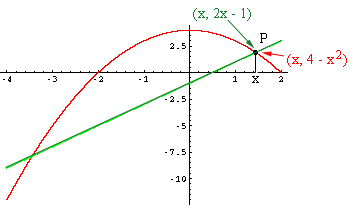Quandaries and Queries JJ Student Secondary Level Questions   Is there a way to find the intersections of these graphs algebraically? x2 + y = 4      &        2x - y = 1 I got (1.45, 1.9) and (-3.45, -7.9) with a graphing calculator. AND THESE... y = 3.29x        &        y = 5.5(x0.5)+ 10000 I got x at 3133 with a graphing calculator. Hi JJ, First write the expressions in the form y = f(x) and y = g(x), that is y = 4 - x2 and y = 2x - 1 I plotted the two functions as you did and gotLook at one of the points where the graphs intersect and call it P. Suppose that the first coordinate of P is x. Since P is on the graph of the first equation its second coordinate is then 4 - x2. Since P is on the graph of the second equation its second coordinate is then 2x - 1.Thus 4 - x2 = 2x - 1, that is x2 + 2x - 5 = 0. Solve for x. (This quadratic doesn't factor so you will need to use the general quadratic formula.) For the second problem the technique is similar. Write the expressions in the form y = f(x) and y = g(x). Set f(x) = g(x) and solve for x. Penny Go to Math Central